# NCERT Solutions for Class 9 Maths Chapter 2 Polynomials| PDF Download

You can find Chapter 2 Polynomials Class 9 Maths NCERT Solutions here that will help you in finding NCERT Solutions for Class 9 Maths Chapter 2 PDF Download easily without wasting your precious time. This will help in developing your problem solving skills and be aware of the concepts. By taking help from these solutions, you can build your own answers for homework and get good marks in the examination.

These solutions are updated according to the latest NCERT Class 9 Maths textbook. These solutions are prerequisites before solving exemplar problems and going for advance Maths Books.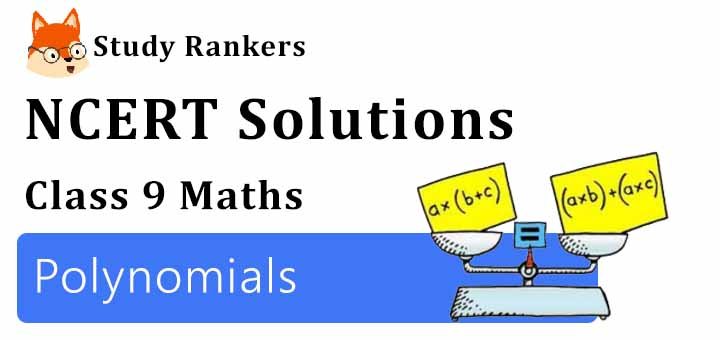Page No: 32

Exercise 2.1

1. Which of the following expressions are polynomials in one variable and which are not? State reasons for your answer.
(i) 4x2 - 3x + 7
(ii) y2 + √2
(iii) 3√t + t2
(iv) y + 2/y
(v) x10 + y3 + t50

(i) 4x2 - 3x + 7
There is only one variable x with whole number power so this polynomial in one variable.

(ii)  y2 + √2
There is only one variable y with whole number power so this polynomial in one variable.

(iii) 3√2 + t
There is only one variable t but in 3√t power of t is 1/2 which is not a whole number so 3√t + t2 is not a polynomial.

(iv) y + 2/y
There is only one variable but 2/y = 2y-1 so the power is not a whole number so y + 2/y is not a polynomial.

(v) x10 + y3 + t50
There are three variable xy and t and there powers are whole number so this polynomial in three variable.

2. Write the coefficients of x2 in each of the following:
(i) 2 + x2 + x
(ii) 2 - x2 + x3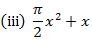(iv) √2x - 1

(i) coefficients of x2 = 1
(ii) coefficients of x2 = -1
(iii) coefficients of x2 = π/2
(iv) coefficients of x2 = 0

3. Give one example each of a binomial of degree 35, and of a monomial of degree 100.

3x35+7 and 4x100

4. Write the degree of each of the following polynomials:
(i) 5x3 + 4x2 + 7x
(ii) 4 – y2
(iii) 5t – √7
(iv) 3

(i) 5x3 has highest power in the given polynomial which power is 3. Therefore, degree of polynomial is 3.

(ii) – y2  has highest power in the given polynomial which power is 2. Therefore, degree of polynomial is 2.

(iii) 5t has highest power in the given polynomial which power is 1. Therefore, degree of polynomial is 1.

(iv) There is no variable in the given polynomial. Therefore, degree of polynomial is 0.

5. Classify the following as linear, quadratic and cubic polynomial:
(i) x2 + x

(ii) x - x3
► Cubic Polynomial

(iii) y + y2 +4

(iv) 1 + x
► Linear Polynomial

(v) 3t
►Linear Polynomial

(vi) r2

(vii) 7x3
► Cubic Polynomial

Page No: 34

Exercise 2.2

1. Find the value of the polynomial at 5x + 4x2 + 3 at

(i) x = 0 (ii) x = - 1 (iii) x = 2

(i) p(x) = 5x + 4x2 + 3
p(0) = 5(0) + 4(0)2 + 3
= 3

(ii) p(x) = 5x + 4x2 + 3
p(-1) = 5(-1) + 4(-1)2 + 3
= -5 + 4(1) + 3 = 2

(iii) p(x) = 5x + 4x2 + 3
p(2) = 5(2) + 4(2)2 + 3
= 10 + 16 + 3 = 29

2. Find p(0), p(1) and p(2) for each of the following polynomials:
(i) p(y) = y2 - y + 1
(ii) p(t) = 2 + t + 2t2 - t3
(iii) p(x) = x3
(iv) p(x) = (x - 1) (x + 1)

(i) p(y) = y2 - y + 1
p(0) = (0)2 - (0) + 1 = 1
p(1) = (1)2 - (1) + 1 = 1
p(2) = (2)2 - (2) + 1 = 3

(ii) p(t) = 2 + t + 2t2 - t3
p(0) = 2 + 0 + 2 (0)2 - (0)3 = 2
p(1) = 2 + (1) + 2(1)2 - (1)3
= 2 + 1 + 2 - 1 = 4
p(2) = 2 + 2 + 2(2)2 - (2)3
= 2 + 2 + 8 - 8 = 4

(iii) p(x) = x3
p(0) = (0)3 = 0
p(1) = (1)3 = 1
p(2) = (2)3 = 8

(iv) p(x) = (x - 1) (x + 1)
p(0) = (0 - 1) (0 + 1) = (- 1) (1) = - 1
p(1) = (1 - 1) (1 + 1) = 0 (2) = 0
p(2) = (2 - 1 ) (2 + 1) = 1(3) = 3

Page No: 35

3. Verify whether the following are zeroes of the polynomial, indicated against them.
(i) p(x) = 3x + 1, x = -1/3
(ii)  p(x) = 5x - π, x = 4/5
(iii) p(x) = x2 - 1, x = 1, -1
(iv) p(x) = (x + 1) (x - 2), x = -1, 2
(v) p(x) = x2 , x = 0(viii) p(x) = 2x + 1, x = 1/2

(i) If x = -1/3 is a zero of polynomial p(x) = 3x + 1 then p(-1/3) should be 0.
At, p(-1/3) = 3(-1/3) + 1 = -1 + 1 = 0
Therefore, x = -1/3 is a zero of polynomial p(x) = 3x + 1.

(ii) If x = 4/5 is a zero of polynomial p(x) = 5x - π then p(4/5) should be 0.
At, p(4/5) = 5(4/5) - π = 4 - π
Therefore, x = 4/5 is not a zero of given polynomial p(x) = 5x - π.

(iii) If x = 1 and x = -1 are zeroes of polynomial p(x) = x2 - 1, then p(1) and p(-1) should be 0.
At, p(1) = (1)2 - 1 = 0 and
At, p(-1) = (-1)2 - 1 = 0
Hence, x = 1 and -1 are zeroes of the polynomial  p(x) = x2 - 1.

(iv) If x = -1 and x = 2 are zeroes of polynomial p(x) = (x +1) (x - 2), then p( - 1) and (2)should be 0.
At, p(-1) = (-1 + 1) (-1 - 2) = 0 (-3) = 0, and
At, p(2) = (2 + 1) (2 - 2) = 3 (0) = 0
Therefore, x = -1 and x = 2 are zeroes of the polynomial p(x) = (x +1) (x - 2).

(v) If x = 0 is a zero of polynomial p(x) = x2, then p(0) should be zero.
Here, p(0) = (0)2 = 0
Hence, x = 0 is a zero of the polynomial p(x) = x2.(viii)  If x = 1/2 is a zero of polynomial p(x) = 2x + 1 then p(1/2) should be 0.
At, p(1/2) = 2(1/2) + 1 = 1 + 1 = 2
Therefore, x = 1/2 is not a zero of given polynomial p(x) = 2x + 1.

4. Find the zero of the polynomial in each of the following cases:
(i) p(x) = x + 5
(ii) p(x) = x - 5
(iii) p(x) = 2x + 5
(iv) p(x) = 3x - 2
(v) p(x) = 3x
(vi) p(x) = axa ≠ 0
(vii) p(x) = cx + d, c ≠ 0, c, are real numbers.

(i) p(x) = x + 5
p(x) = 0
x + 5 = 0
x = -5
Therefore, x = -5 is a zero of polynomial p(x) = x + 5 .

(ii) p(x) = x - 5
p(x) = 0
x - 5 = 0
x = 5
Therefore, x = 5 is a zero of polynomial p(x) = x - 5.

(iii) p(x) = 2x + 5
p(x) = 0
2x + 5 = 0
2x = -5
x = -5/2
Therefore, x = -5/2 is a zero of polynomial p(x) = 2x + 5.

(iv) p(x) = 3x - 2
p(x) = 0
3x - 2 = 0
x = 2/3
Therefore, x = 2/3 is a zero of polynomial p(x) = 3x - 2.

(v) p(x) = 3x
p(x) = 0
3x = 0
x = 0
Therefore, x = 0 is a zero of polynomial p(x) = 3x.

(vi) p(x) = ax
p(x) = 0
ax = 0
= 0
Therefore, x = 0 is a zero of polynomial p(x) = ax.

(vii) p(x) = cx + d
p(x) = 0
cx + d = 0
x = -d/c
Therefore, x = -d/c is a zero of polynomial p(x) = cx + d.

Page No: 40

Exercises 2.3

1. Find the remainder when x3 + 3x2 + 3x + 1 is divided by
(i) x + 1
(ii) x - 1/2
(iii) x
(iv) x + π
(v) 5 + 2x

(i) x + 1
By long division,Therefore, the remainder is 0.

(ii) x - 1/2
By long division,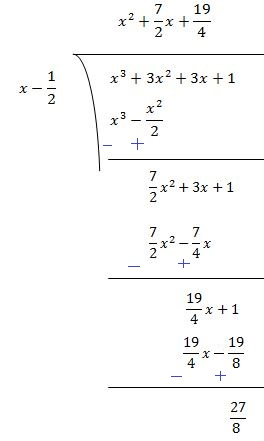Therefore, the remainder is 27/8.

(iii) x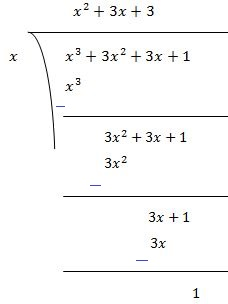Therefore, the remainder is 1.

(iv) x + π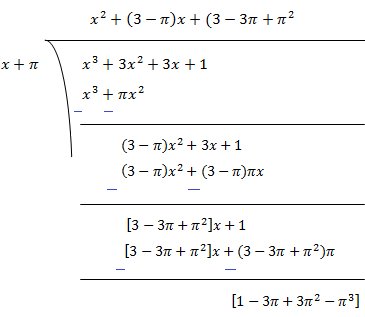Therefore, the remainder is [1 - 3π + 3π2 - π3].

(v) 5 + 2xTherefore, the remainder is -27/8.

2. Find the remainder when x3 - ax2 + 6x - a is divided by x - a.

By Long Division,Therefore, remainder obtained is 5when x3 - ax2 + 6x - a is divided by x - a.

3. Check whether 7 + 3x is a factor of 3x3 + 7x.

We have to divide 3x3 + 7by 7 + 3x. If remainder comes out to be 0 then 7 + 3x will be a factor of 3x3 + 7x.
By Long Division,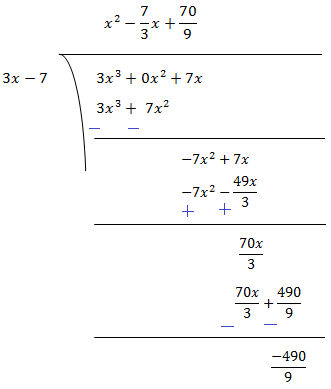As remainder is not zero so 7 + 3x is not a factor of 3x3 + 7x.

Page No: 43

Exercise 2.4

1. Determine which of the following polynomials has (x + 1) a factor:
(i) x3 + x2 + x + 1
(ii) x4 + x3 + x2 + x + 1
(iii) x4 + 3x3 + 3x2 + x + 1
(iv) x3 - x2 - (2 + √2)x + √2

(i) If (x + 1) is a factor of p(x) = x3 + x2 + x + 1, p(-1) must be zero.
Here, p(x) = x3 + x2 + x + 1
p(-1) = (-1)3 + (-1)2 + (-1) + 1
= -1 + 1 - 1 + 1 = 0
Therefore, x + 1 is a factor of this polynomial

(ii) If (x + 1) is a factor of p(x) = x4 + x3 + x2 + x + 1, p(-1) must be zero.
Here, p(x) = x4 + x3 + x2 + x + 1
p(-1) = (-1)4 + (-1)3 + (-1)2 + (-1) + 1
= 1 - 1 + 1 - 1 + 1 = 1
As, p(-1) ≠ 0
Therefore, x + 1 is not a factor of this polynomial

(iii)If (x + 1) is a factor of polynomial p(x) = x4 + 3x3 + 3x2 + x + 1, p(- 1) must be 0.
p(-1) = (-1)4 + 3(-1)3 + 3(-1)2 + (-1) + 1
= 1 - 3 + 3 - 1 + 1 = 1
As, p(-1) ≠ 0
Therefore, x + 1 is not a factor of this polynomial.

(iv) If (x + 1) is a factor of polynomial
p(x) = x3 - x2 - (2 + √2)x + √2p(- 1) must be 0.
p(-1) =  (-1)3 -  (-1)2 -  (2 + √2) (-1) + √2
= -1 - 1 + 2 + √2 + √2
=2√2
As, p(-1) ≠ 0
Therefore,, x + 1 is not a factor of this polynomial.

2. Use the Factor Theorem to determine whether g(x) is a factor of p(x) in each of the following cases:
(i) p(x) = 2x3 + x2 - 2x - 1, g(x) = x + 1
(ii) p(x) = x3 + 3x2 + 3x + 1, g(x) = x + 2
(iii) p(x) = x3 - 4 x2 + x + 6, g(x) = x - 3

(i) If g(x) = x + 1 is a factor of given polynomial p(x), p(- 1) must be zero.
p(x) = 2x3 + x2 - 2x - 1
p(- 1) = 2(-1)3 + (-1)2 - 2(-1) - 1
= 2(- 1) + 1 + 2 - 1 = 0
Hence, g(x) = x + 1 is a factor of given polynomial.

(ii) If g(x) = x + 2 is a factor of given polynomial p(x), p(- 2) must be 0.
p(x) = x3 +3x2 + 3x + 1
p(-2) = (-2)3 + 3(- 2)2 + 3(- 2) + 1
= -8 + 12 - 6 + 1
= -1
As, p(-2) ≠ 0
Hence g(x) = x + 2 is not a factor of given polynomial.

(iii) If g(x) = x - 3 is a factor of given polynomial p(x), p(3) must be 0.
p(x) = x3 - 4x2 + x + 6
p(3) = (3)3 - 4(3)2 + 3 + 6
= 27 - 36 + 9 = 0
Therefore,, g(x) = x - 3 is a factor of given polynomial.

Page No: 44

3. Find the value of k, if x - 1 is a factor of p(x) in each of the following cases:(i) p(x) = x2 + x + k
(ii) p(x) = 2x2 + kx +  √2
(iii) p(x) = kx2 - √2x + 1
(iv) p(x) = kx2 - 3x + k

(i) If x - 1 is a factor of polynomial p(x) = x2 + x + k, then
p(1) = 0
⇒ (1)2 + 1 + k = 0
⇒ 2 + k = 0
⇒ k = - 2
Therefore, value of k is -2.

(ii) If x - 1 is a factor of polynomial p(x) = 2x2 + kx +  √2, then
p(1) = 0
⇒ 2(1)2 + k(1) + √2 = 0
⇒ 2 + k + 2 = 0
⇒ k = -2 - √2 = -(2 + √2)
Therefore, value of k is -(2 + √2).

(iii) If x - 1 is a factor of polynomial p(x) = kx2 - √2x + 1, then
p(1) = 0
⇒ k(1)2 - √2(1) + 1 = 0
⇒ k - √2 + 1 = 0
⇒ k = √2 - 1
Therefore, value of k is √2 - 1.

(iv) If x - 1 is a factor of polynomial p(x) = kx2 - 3x + k, then
p(1) = 0
⇒ k(1)2 + 3(1) + k = 0
⇒ k - 3 + k = 0
⇒ 2k - 3 = 0
⇒ k = 3/2
Therefore, value of k is 3/2.

4. Factorise:
(i) 12x2 + 7x + 1
(ii) 2x2 + 7x + 3
(iii) 6x2 + 5x - 6
(iv) 3x2 - x - 4

(i) 12x2 + 7x + 1
= 12x2 - 4x - 3x+ 1
= 4x (3x - 1) - 1 (3x - 1)
= (3x - 1) (4x - 1)

(ii) 2x2 + 7x + 3
= 2x2 + 6x + + 3
= 2x (x + 3) + 1 (x + 3)
(x + 3) (2+ 1)

(iii) 6x2 + 5x - 6
= 6x2 + 9- 4x - 6
= 3x (2x + 3) - 2 (2x + 3)
= (2x + 3) (3x - 2)

(iv) 3x2 - x - 4
= 3x2 - 4+ 3x - 4
x (3x - 4) + 1 (3x - 4)
= (3x - 4) (x + 1)

5. Factorise:
(i) x3 - 2x2 - x + 2
(ii) x3 - 3x2 - 9x - 5
(iii) x3 + 13x2 + 32x + 20
(iv) 2y3 + y2 - 2y - 1

(i) Let p(x) = x3 - 2x2 - x + 2
Factors of 2 are ±1 and ± 2
By trial method, we find that
p(1) = 0
So, (x+1) is factor of p(x)
Now,
p(x) = x3 - 2x2 - x + 2
p(-1) = (-1)3 - 2(-1)2 - (-1) + 2 = -1 -2 + 1 + 2 = 0
Therefore, (x+1) is the factor of  p(x)Now, Dividend = Divisor × Quotient + Remainder
(x+1) (x2 - 3x + 2)
= (x+1) (x2 - x - 2x + 2)
= (x+1) {x(x-1) -2(x-1)}
= (x+1) (x-1) (x+2)

(ii) Let p(x) = x3 - 3x2 - 9x - 5
Factors of 5 are ±1 and ±5
By trial method, we find that
p(5) = 0
So, (x-5) is factor of p(x)
Now,
p(x) = x3 - 2x2 - x + 2
p(5) = (5)3 - 3(5)2 - 9(5) - 5 = 125 - 75 - 45 - 5 = 0
Therefore, (x-5) is the factor of  p(x)Now, Dividend = Divisor × Quotient + Remainder
(x-5) (x2 + 2x + 1)
= (x-5) (x2 + x + x + 1)
(x-5) {x(x+1) +1(x+1)}
(x-5) (x+1) (x+1)

(iii) Let p(x) = x3 + 13x2 + 32x + 20
Factors of 20 are ±1, ±2, ±4, ±5, ±10 and ±20
By trial method, we find that
p(-1) = 0
So, (x+1) is factor of p(x)
Now,
p(x) =  x3 + 13x2 + 32x + 20
p(-1) = (-1)3 + 13(-1)2 + 32(-1) + 20 = -1 + 13 - 32 + 20 = 0
Therefore, (x+1) is the factor of  p(x)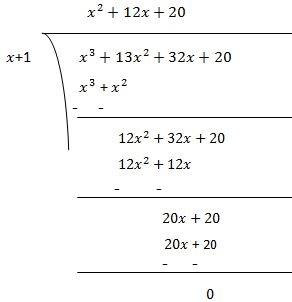Now, Dividend = Divisor × Quotient + Remainder
(x+1) (x2 + 12x + 20)
= (x+1) (x2 + 2x + 10x + 20)
(x-5) {x(x+2) +10(x+2)}
(x-5) (x+2) (x+10)

(iv) Let p(y) = 2y3 + y2 - 2y - 1
Factors of ab = 2× (-1) = -2 are ±1 and ±2
By trial method, we find that
p(1) = 0
So, (y-1) is factor of p(y)
Now,
p(y) =  2y3 + y2 - 2y - 1
p(1) = 2(1)3 + (1)2 - 2(1) - 1 = 2 +1 - 2 - 1 = 0
Therefore, (y-1) is the factor of  p(y)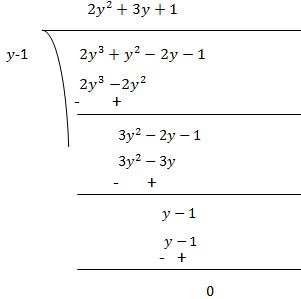Now, Dividend = Divisor × Quotient + Remainder
(y-1) (2y2 + 3y + 1)
(y-1) (2y2 + 2y + y + 1)
(y-1) {2y(y+1) +1(y+1)}
(y-1) (2y+1) (y+1)

Page No: 48

Exercise 2.5

1. Use suitable identities to find the following products:
(i) (x + 4) (x + 10)                     (ii) (x + 8) (x – 10)                      (iii) (3x + 4) (3x – 5)
(iv) (y+ 3/2) (y- 3/2)             (v) (3 - 2x) (3 + 2x)

(i) Using identity, (+ a) (x + b) = x2 + (a + b) x + ab
In (x + 4) (x + 10), a = 4 and b = 10
Now,
(x + 4) (x + 10) = x2 + (4 + 10)x + (4 × 10)
= x2 + 14x + 40

(ii) (x + 8) (x – 10)
Using identity, (+ a) (x + b) = x2 + (a + b) x + ab
Here, a = 8 and b = –10
(x + 8) (x – 10) = x2 + {8 +(– 10)}x + {8×(– 10)}
= x2 + (8 – 10)x – 80
= x2 – 2x – 80

(iii) (3x + 4) (3x – 5)
Using identity, (+ a) (x + b) = x2 + (a + b) x + ab
Here, x = 3x , a = 4 and b = -5
(3x + 4) (3x – 5) = (3x2 + {4 + (-5)}3x + {4×(-5)}
= 9x2 + 3x(4 - 5) - 20
= 9x2 - 3x - 20

(iv) (y+ 3/2) (y- 3/2)
Using identity, (+ y) (x -y) = x2 - y2
Here, x = y2 and y = 3/2
(y+ 3/2) (y- 3/2) = (y2)- (3/2)2
y4 - 9/4

(v) (3 - 2x) (3 + 2x)
Using identity, (+ y) (x -y) = x2 - y2
Here, x = 3 and y = 2x
(3 - 2x) (3 + 2x) = 32 - (2x)2
=  9 - 4x2

2. Evaluate the following products without multiplying directly:
(i) 103 × 107               (ii) 95 × 96               (iii) 104 × 96

(i) 103 × 107 = (100 + 3) (100 + 7)
Using identity, (+ a) (x + b) = x2 + (a + b) x + ab
Here, x = 100, a = 3 and b = 7
103 × 107 = (100 + 3) (100 + 7) = (100)2 + (3 + 7)10 + (3 × 7)
= 10000 + 100 + 21
= 10121

(ii) 95 × 96 = (90 + 5) (90 + 4)
Using identity, (+ a) (x + b) = x2 + (a + b) x + ab
Here, x = 90, a = 5 and b = 4
95 × 96 = (90 + 5) (90 + 4) = 902 + 90(5 + 6) + (5 × 6)
= 8100 + (11 × 90) + 30
= 8100 + 990 + 30 = 9120

(iii) 104 × 96 = (100 + 4) (100 - 4)
Using identity, (+ y) (x -y) = x2 - y2
Here, x = 100 and y = 4
104 × 96 = (100 + 4) (100 - 4) = (100)2 - (4)= 10000 - 16 = 9984

3. Factorise the following using appropriate identities:
(i) 9x2 + 6xy + y2                 (ii) 4y2 - 4y + 1              (iii) xy2/100

(i) 9x2 + 6xy + y2  = (3x) 2 + (2×3x×y) + y2
Using identity, (a + b)2 = a2 + 2ab + b2
Here, a = 3x and b = y
9x2 + 6xy + y2  = (3x) 2 + (2×3x×y) + y= (3x + y)= (3x + y) (3x + y)

(ii) 4y2 - 4y + 1 = (2y)2 - (2×2y×1) + 12
Using identity, (a - b)2 = a2 - 2ab + b2
Here, a = 2y and b = 1
4y2 - 4y + 1 = (2y)2 - (2×2y×1) + 1= (2y - 1)= (2y - 1) (2y - 1)

(iii) x- y2/100 = x- (y/10)2
Using identity, a2 - b2 = (a + b) (a - b)
Here, a = x and b = (y/10)
x- y2/100 = x- (y/10)= (x - y/10) (x + y/10)

Page No: 49

4. Expand each of the following, using suitable identities:
(i) (x + 2y + 4z)2                     (ii) (2x – y + z)2                    (iii) (–2x + 3y + 2z)2
(iv) (3a – 7b – c)2                         (v) (–2x + 5y – 3z)2                   (vi) [1/4 a - 1/2 b + 1]2

(i) (x + 2y + 4z)2
Using identity, (a + b + c)= a2 + b2 + c2 + 2ab + 2bc + 2ca
Here, a = x, b = 2and c = 4z
(x + 2y + 4z)x2 + (2y)2 + (4z)2 + (2×x×2y) + (2×2y×4z) + (2×4z×x)
= x2 + 4y2 + 16z2 + 4xy + 16yz + 8xz

(ii)  (2x – y + z)2
Using identity, (a + b + c)= a2 + b2 + c2 + 2ab + 2bc + 2ca
Here, a = 2x, b = -and c = z
(2x – y + z)= (2x)2 + (-y)2 + z2 + (2×2x×-y) + (2×-y×z) + (2×z×2x)
= 4x2 + y2 + z2 - 4xy - 2yz + 4xz

(iii) (–2x + 3y + 2z)2
Using identity, (a + b + c)= a2 + b2 + c2 + 2ab + 2bc + 2ca
Here, a = -2x, b = 3and c = 2z
(–2x + 3y + 2z)(-2x)2 + (3y)2 + (2z)2 + (2×-2x×3y) + (2×3y×2z) + (2×2z×-2x)
= 4x2 + 9y2 + 4z2 - 12xy + 12yz - 8xz

(iv) (3a – 7b – c)2
Using identity, (a + b + c)= a2 + b2 + c2 + 2ab + 2bc + 2ca
Here, a = 3a, b = -7b and c = -c
(3a – 7b – c)2 (3a)2 + (-7b)2 + (-c)2 + (2×3a×-7b) + (2×-7b×-c) + (2×-c×3a)
= 9a2 + 49b2 + c2 - 42ab + 14bc - 6ac

(v) (–2x + 5y – 3z)2
Using identity, (a + b + c)= a2 + b2 + c2 + 2ab + 2bc + 2ca
Here, a = -2x, b = 5y and c = -3z
(–2x + 5y – 3z)2 (-2x)2 + (5y)2 + (-3z)2 + (2×-2x×5y) + (2×5y×-3z) + (2×-3z×-2x)
= 4x2 + 25y2 + 9z2 - 20xy - 30yz + 12xz

(vi) [1/4 a - 1/2 b + 1]2
Using identity, (a + b + c)= a2 + b2 + c2 + 2ab + 2bc + 2ca
Here, a = 1/4 a, b = -1/2 b and c = 1
[1/4 a - 1/2 b + 1]2 (1/4 a)2 + (-1/2 b)2 + 12 + (2×1/4 a×-1/2 b) + (2×-1/2 b×1) + (2×1×1/4 a)
= 1/16 a2 + 1/4 b2 + 1 - 1/4 ab - b + 1/2 a

5. Factorise:
(i) 4x2 + 9y2 + 16z2 + 12xy - 24yz - 16xz
(ii) 2x2 + y2 + 8z2 - 2√2 xy + 4√2 yz - 8xz

(i) 4x2 + 9y2 + 16z2 + 12xy - 24yz - 16xz
Using identity, (a + b + c)= a2 + b2 + c2 + 2ab + 2bc + 2ca
4x2 + 9y2 + 16z2 + 12xy - 24yz - 16xz
= (2x)2 + (3y)2 + (-4z)2 + (2×2x×3y) + (2×3y×-4z) + (2×-4z×2x)
= (2x + 3y - 4z)2
(2x + 3y - 4z) (2x + 3y - 4z)

(ii) 2x2 + y2 + 8z2 - 2√2 xy + 4√2 yz - 8xz
Using identity, (a + b + c)= a2 + b2 + c2 + 2ab + 2bc + 2ca
2x2 + y2 + 8z2 - 2√2 xy + 4√2 yz - 8xz
= (-√2x)2 + (y)2 + (2√2z)2 + (2×-√2x×y) + (2×y×2√2z) + (2×2√2z×-√2x)
(-√2x + y + 2√2z)2
(-√2x + y + 2√2z) (-√2x + y + 2√2z)

6. Write the following cubes in expanded form:
(i) (2x + 1)3                 (ii) (2a – 3b)3                (iii) [3/2 x + 1]3           (iv) [x - 2/3 y]3

(i) (2x + 1)3
Using identity, (a + b)= a3 + b3 + 3ab(a + b)
(2x + 1)= (2x)3 + 13 + (3×2x×1)(2x + 1)
= 8x3 + 1 + 6x(2x + 1)
8x3 + 12x2 + 6x + 1

(ii) (2a – 3b)3
Using identity, (a - b)= a3 - b3 - 3ab(a - b)
(2a – 3b)= (2a)3 - (3b)3 - (3×2a×3b)(2a - 3b)
= 8a3 - 27b3 - 18ab(2a - 3b)
8a3 - 27b3 - 36a2b + 54ab2

(iii) [3/2 x + 1]3
Using identity, (a + b)= a3 + b3 + 3ab(a + b)
[3/2 x + 1]= (3/2 x)3 + 13 + (3×3/2 x×1)(3/2 x + 1)
= 27/8 x+ 1 + 9/2 x(3/2 x + 1)
27/8 x+ 1 + 27/4 x2 + 9/2 x
27/8 x+ 27/4 x2 + 9/2 x + 1

(iv) [x - 2/3 y]3
Using identity, (a - b)= a3 - b3 - 3ab(a - b)
[x - 2/3 y]3 = (x)3 - (2/3 y)3 - (3×x×2/3 y)(x - 2/3 y)
= x3 - 8/27y3 - 2xy(x - 2/3 y)
x3 - 8/27y3 - 2x2y + 4/3xy2

7. Evaluate the following using suitable identities:
(i) (99)3            (ii) (102)3             (iii) (998)3

(i) (99)3 = (100 - 1)3
Using identity, (a - b)= a3 - b3 - 3ab(a - b)
(100 - 1)= (100)3 - 13 - (3×100×1)(100 - 1)
= 1000000 - 1 - 300(100 - 1)
= 1000000 - 1 - 30000 + 300
= 970299

(ii) (102)3 = (100 + 2)3
Using identity, (a + b)= a3 + b3 + 3ab(a + b)
(100 + 2)= (100)3 + 23 + (3×100×2)(100 + 2)
= 1000000 + 8 + 600(100 + 2)
= 1000000 + 8 + 60000 + 1200
= 1061208

(iii) (998)3
Using identity, (a - b)= a3 - b3 - 3ab(a - b)
(1000 - 2)= (1000)3 - 23 - (3×1000×2)(1000 - 2)
= 100000000 - 8 - 6000(1000 - 2)
= 100000000 - 8- 600000 + 12000
= 994011992

8. Factorise each of the following:
(i) 8a3 + b3 + 12a2b + 6ab2                           (ii) 8a3 - b3 - 12a2b + 6ab2
(iii) 27 - 125a3 - 135a + 225a2                      (iv) 64a3 - 27b3 - 144a2b + 108ab2
(v) 27p3 - 1/216 - 9/2 p2 + 1/4 p

(i) 8a3 + b3 + 12a2b + 6ab2
Using identity, (a + b)= a3 + b3 + 3a2b + 3ab2
8a3 + b3 + 12a2b + 6ab2
= (2a)3 + b3 + 3(2a)2b + 3(2a)(b)2
= (2a + b)3
= (2a + b)(2a + b)(2a + b)

(ii) 8a3 - b3 - 12a2b + 6ab2
Using identity, (a - b)= a3 - b3 - 3a2b + 3ab2
8a3 - b3 - 12a2b + 6ab2= (2a)3 - b3 - 3(2a)2b + 3(2a)(b)2
= (2a - b)3
= (2a - b)(2a - b)(2a - b)

(iii) 27 - 125a3 - 135a + 225a2
Using identity, (a - b)= a3 - b3 - 3a2b + 3ab2
27 - 125a3 - 135a + 225a2= 33 - (5a)3 - 3(3)2(5a) + 3(3)(5a)2
= (3 - 5a)3
(3 - 5a)(3 - 5a)(3 - 5a)

(iv) 64a3 - 27b3 - 144a2b + 108ab2
Using identity, (a - b)= a3 - b3 - 3a2b + 3ab2
64a3 - 27b3 - 144a2b + 108ab2= (4a)3 - (3b)3 - 3(4a)2(3b) + 3(4a)(3b)2
= (4a - 3b)3
= (4a - 3b)(4a - 3b)(4a - 3b)

(v) 27p3 - 1/216 - 9/2 p2 + 1/4 p

Using identity, (a - b)= a3 - b3 - 3a2b + 3ab2
27p3 - 1/216 - 9/2 p2 + 1/4 p
(3p)3 - (1/6)3 - 3(3p)2(1/6) + 3(3p)(1/6)2
= (3p - 1/6)3
= (3p - 1/6)(3p - 1/6)(3p - 1/6)

9. Verify : (i) x3 + y3 = (x + y) (x2 - xy + y2)             (ii) x3 - y3 = (x - y) (x2 + xy + y2)

(i) x3 + y3 = (x + y) (x2 - xy + y2)
We know that,
(x + y)= x3 + y3 + 3xy(x + y)
⇒ x3 + y= (x + y)- 3xy(x + y)
⇒ x3 + y= (x + y)[(x + y)2 - 3xy]                  {Taking (x+y) common}
⇒ x3 + y= (x + y)[(x2 + y+ 2xy) - 3xy]
⇒ x3 + y= (x + y)(x2 + y- xy)

(ii) x3 - y3 = (x - y) (x2 + xy + y2 )
We know that,
(x - y)= x3 - y3 - 3xy(x - y)
⇒ x3 - y= (x - y)+ 3xy(x - y)
⇒ x3 + y= (x - y)[(x - y)2 + 3xy]                     {Taking (x-y) common}
⇒ x3 + y= (x - y)[(x2 + y- 2xy) + 3xy]
⇒ x3 + y= (x + y)(x2 + y+ xy)

10. Factorise each of the following:
(i) 27y3 + 125z3                     (ii) 64m3 - 343n3

(i) 27y3 + 125z3
Using identity, x3 + y3 = (x + y) (x2 - xy + y2)
27y3 + 125z3 = (3y)3 + (5z)3
(3y + 5z) {(3y)2 - (3y)(5z) + (5z)2}
= (3y + 5z) (9y2 - 15yz + 25z)2

(ii) 64m3 - 343n3
Using identity, x3 - y3 = (x - y) (x2 + xy + y2 )
64m3 - 343n3 = (4m)3 - (7n)3
(4m + 7n) {(4m)2 + (4m)(7n) + (7n)2}
= (4m + 7n) (16m2 + 28mn + 49n)2

11. Factorise : 27x3 + y3 + z3 - 9xyz

27x3 + y3 + z3 - 9xyz = (3x)3 + y3 + z3 - 3×3xyz
Using identity, x3 + y3 + z3 - 3xyz = (x + y + z)(x2 + y2 + z2 - xy - yz - xz)
27x3 + y3 + z3 - 9xyz
(3x + y + z) {(3x)2 + y2 + z2 - 3xy - yz - 3xz}
(3x + y + z) (9x2 + y2 + z2 - 3xy - yz - 3xz)

12. Verify that: x3 + y3 + z3 - 3xyz = 1/2(x + y + z) [(x - y)+ (y - z)+ (z - x)2]

We know that,
x3 + y3 + z3 - 3xyz = (x + y + z)(x2 + y2 + z2 - xy - yz - xz)
x3 + y3 + z3 - 3xyz = 1/2×(x + y + z) 2(x2 + y2 + z2 - xy - yz - xz)
= 1/2(x + y + z) (2x2 + 2y2 + 2z2 - 2xy - 2yz - 2xz)
1/2(x + y + z) [(x2 + y2 -2xy) + (y+ z2 - 2yz) + (x2 + z- 2xz)]
= 1/2(x + y + z) [(x - y)+ (y - z)+ (z - x)2]

13. If x + y + z = 0, show that x3 + y3 + z3 = 3xyz.

We know that,
x3 + y3 + z3 - 3xyz = (x + y + z)(x2 + y2 + z2 - xy - yz - xz)
Now put (x + y + z) = 0,
x3 + y3 + z3 - 3xyz = (0)(x2 + y2 + z2 - xy - yz - xz)
⇒ x3 + y3 + z3 - 3xyz = 0

14. Without actually calculating the cubes, find the value of each of the following:
(i) (-12)3 + (7)3 + (5)3
(ii) (28)3 + (–15)3 + (-13)3

(i) (-12)3 + (7)3 + (5)3
Let x = -12, y = 7 and z = 5
We observed that, x + y + z = -12 + 7 + 5 = 0

We know that if,
x + y + z = 0, then x3 + y3 + z3 = 3xyz
(-12)3 + (7)3 + (5)3 = 3(-12)(7)(5) = -1260

(ii) (28)3 + (–15)3 + (-13)3
Let x = 28, y = -15 and z = -13
We observed that, x + y + z = 28 - 15 - 13 = 0

We know that if,
x + y + z = 0, then x3 + y3 + z3 = 3xyz
(28)3 + (–15)3 + (-13)3 = 3(28)(-15)(-13) = 16380

15. Give possible expressions for the length and breadth of each of the following rectangles, in which their areas are given:
(i) Area : 25a2 - 35a + 12
(ii) Area : 35 y2 + 13y - 12

(i) Area : 25a2 - 35a + 12

Since, area is product of length and breadth therefore by factorizing the given area, we can know the length and breadth of rectangle.
25a2 - 35a + 12
25a2 - 15a -20a + 12
= 5a(5a - 3) - 4(5a - 3)
(5a - 4)(5a - 3)
Possible expression for length = 5a - 4
Possible expression for breadth = 5a - 3

(ii) Area : 35 y2 + 13y - 12
35 y2 + 13y - 12
35y2 - 15y + 28y - 12
= 5y(7y - 3) + 4(7y - 3)
(5y + 4)(7y - 3)
Possible expression for length = (5y + 4)
Possible expression for breadth (7y - 3)

Page No: 50

16. What are the possible expressions for the dimensions of the cuboids whose volumes are given below? (i) Volume : 3x2 - 12x
(ii) Volume : 12ky2 + 8ky - 20k

(i) Volume : 3x2 - 12
Since, volume is product of length, breadth and height therefore by factorizing the given volume, we can know the length, breadth and height of the cuboid.
3x2 - 12x
= 3x(x - 4)
Possible expression for length = 3
Possible expression for height (x - 4)

(ii) Volume : 12ky2 + 8ky - 20k
Since, volume is product of length, breadth and height therefore by factorizing the given volume, we can know the length, breadth and height of the cuboid.
12ky2 + 8ky - 20k
= 4k(3y2 + 2y - 5)
= 4k(3y2 +5y - 3y - 5)
4k[y(3y +5) - 1(3y + 5)]
4k (3y +5) (y - 1)
Possible expression for length = 4k
Possible expression for breadth (3y +5)
Possible expression for height (y - 1)

## NCERT Solutions for Class 9 Maths Chapter 2 Polynomials

We have already understood about algebraic expressions in previous class and in this chapter we will studying about a particular algebraic expression, Polynomial. What is Polynomial? An algebraic expression containing one or more than one terms is called Polynomial. We will study the Remainder Theorem, Factor Theorem and algebraic identities and their use in factorisation of polynomials.

• Polynomials in one Variable: We will discuss linear, quadratic and cubic polynomial in this section briefly.

• Zeroes of a Polynomial: You will know how to find the zero of the polynomial in given cases.

• Remainder Theorem: Let p(x) be any polynomial of degree greater than or equal to one and let a be any real number. If p(x) is divided by the linear polynomial x – a, then the remainder is p(a).

• Factorisation of Polynomials: If p(x) is a polynomial of degree n > 1 and a is any real number, then (i) x – a is a factor of p(x), if p(a) = 0, and (ii) p(a) = 0, if x – a is a factor of p(x).

• Algebraic Identities: We will be solving questions of the exercise based on the given four identities:
Identity I: (x + y)2 = x2 + 2xy + y2
Identity II: (x – y)2 = x2 – 2xy + y2
Identity III : x2 – y2 = (x + y) (x – y)
Identity IV : (x + a) (x + b) = x2 + (a + b)x + ab

You will find total 5 exercises in the whole chapter that are very helpful in building your basic concepts. Studyrankers experts have prepared exercisewise answers of every question for Chapter 2 Polynomials NCERT Solutions Class 9 which you can find below.

These NCERT Solutions for Class 9 Maths prepared by Studyrankers will help you in revising whole topics in less time and improving skills.

### NCERT Solutions for Class 9 Maths Chapters:

 Chapter 1 Number Systems Chapter 3 Coordinate Geometry Chapter 4 Linear Equations in Two Variables Chapter 5 Introduction to Euclid's Geometry Chapter 6 Lines and Angles Chapter 7 Triangles Chapter 8 Quadrilaterals Chapter 9 Areas of Parallelograms and Triangles Chapter 10 Circles Chapter 11 Constructions Chapter 12 Heron's Formula Chapter 13 Surface Areas And Volumes Chapter 14 Statistics Chapter 15 Probability

FAQ on Chapter 2 Polynomials

#### How can I download Chapter 2 Polynomials Class 9 NCERT Solutions PDF

Chapter 2 Polynomials Class 9 Maths NCERT Solutions PDF download is very useful in understanding the basic concepts embedded in the chapter. It is very essential to solve every question before moving further to any other supplementary books.

#### What do you mean by Algebraic Expressions?

A combination of constants and variables, connected by some or all the basic operations +, –, ×, ÷ is called an algebraic expression.

#### What is the coefficient of a zero polynomial?

The coefficient of a zero polynomial is 1.